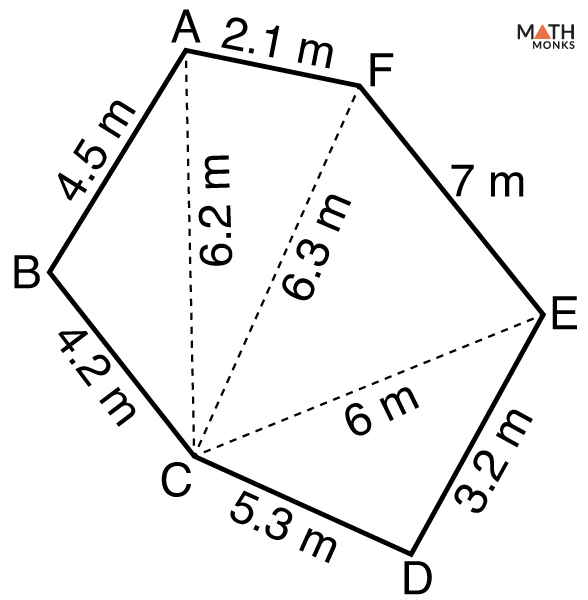# Irregular Hexagon

A hexagon is regular and irregular based on the measure of its sides and angles.

Let us see how an irregular hexagon is different from a regular one.

A regular hexagon has 6 equal sides, 6 equal interior angles each of 120°. The total sum of its interior angles is 720°, 6 exterior angles, each of 60°, 9 diagonals, and 6 lines of symmetry.

In contrast, an irregular hexagon does not satisfy the above features. As the term ‘irregular‘ suggests, an irregular hexagon is a 6-sided polygon with unequal measures of sides and angles.

The properties of an irregular hexagon are:

1. Has 6 unequal sides
2. The interior angles are not equal but their sum is 720°
3. The exterior angles are not equal but their sum will be 360°
4. Cannot be inscribed in a circle
5. A circle cannot be inscribed in it
6. Has 9 unequal diagonals
7. Can be convex or a concave
8. Diagonals do not intersect at a common point
9. Does not have any line of symmetry

We can find the area and perimeter of a regular hexagon very easily. Let us see how to calculate the area and perimeter of an irregular hexagon.

## Area

There is no definite formula to find the area of an irregular hexagon.

Let us solve an example to understand the concept better.Find the area of an irregular hexagon shaped land shown below. 6 of its sides and 3 of its diagonals are known.

++++++

Solution:

Area of the hexagon ABCDEF = Area of ΔABC + Area of ΔACF+ Area of ΔFCE + Area of ΔECD
Here we will use Heron’s formula to find the area of each triangle.
Area (A) = √s(s – a)( s– b)(s – c), here s = ½ (a + b + c); a, b, and c are the 3 sides of any triangle
In ΔABC, s = ½ (a + b + c) here a = 4.5 m, b = 4.2 m, c = 6.2 m
= ½ (4.5  + 4.2  + 6.2)
= 7.45 m
Area (A)ΔABC = √s(s – a)( s– b)(s – c), here s = 7.45 m
= √7.45(7.45 – 4.5)(7.45 – 4.2)(7.45 – 6.2)
≈ 9.45 sq. m
In ΔACF, s = ½ (a + b + c), a = 6.2 m, b = 6.3 m, c = 2.1 m
= ½ (6.2  + 6.3  + 2.1)
= 7.3 m
Area (A)ΔACF = √s(s – a)( s– b)(s – c), here s = 7.3 m
= √7.3(7.3 – 6.2)(7.3 – 6.3)(7.3 – 2.1)
= 6.46 sq. m
In ΔFCE, s = ½ (a + b + c), a = 6.3 m, b = 6 m, c = 7 m
= ½ (6.3 + 6 + 7)
= 9.65 m
Area (A)ΔFCE = √s(s – a)( s– b)(s – c), here s = 9.65 m
= √9.65(9.65 – 6.3)( 9.65 -6)( 9.65 – 7)
= 17.68 sq. m
In ΔECD, s = ½ (a + b + c), a = 6 m, b  = 5.3 m, c = 3.2 m
= ½ (6 + 5.3 + 3.2)
= 7.25 m
Area (A)ΔECD = √s(s – a)( s– b)(s – c), here s = 7.25 m
= √7.25(7.25 – 6)(7.25 – 5.3)(7.25 – 3.2)
≈ 8.46 sq. m
Area of the hexagon ABCDEF = AΔABC + AΔACF + AΔFCE + AΔECD
= 9.45 + 6.46 + 17.68 + 8.46
= 42.05 sq. m

## Perimeter

There is no definite formula to find the perimeter of an irregular hexagon. We can find it when all the sides are known and add them together.

Let us solve an example to understand the concept better.

Find the perimeter of the hexagon with side lengths 4 cm, 12 cm, 11 cm, 7 cm, 6 cm, and 5 cm.

Solution:

As we know,
P = 4 + 12+ 11 + 7 + 6 + 5
= 45 cm